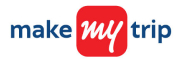New update is available. Click here to update.

# Maximum sum of non-adjacent elements

Medium0/80
15 mins
85 %260 upvotes+14 more

## Problem Statement

#### You are given an array/list of ‘N’ integers. You are supposed to return the maximum sum of the subsequence with the constraint that no two elements are adjacent in the given array/list.

##### Note:
``````A subsequence of an array/list is obtained by deleting some number of elements (can be zero) from the array/list, leaving the remaining elements in their original order.
``````
Detailed explanation ( Input/output format, Notes, Images )
##### Constraints:
``````1 <= T <= 500
1 <= N <= 1000
0 <= ARR[i] <= 10^5

Where 'ARR[i]' denotes the 'i-th' element in the array/list.

Time Limit: 1 sec.
``````
##### Sample Input 1:
``````2
3
1 2 4
4
2 1 4 9
``````
##### Sample Output 1:
``````5
11
``````
##### Explanation to Sample Output 1:
``````In test case 1, the sum of 'ARR' & 'ARR' is 5 which is greater than 'ARR' which is 2 so the answer is 5.

In test case 2, the sum of 'ARR' and 'ARR' is 6, the sum of 'ARR' and 'ARR' is 10, and the sum of 'ARR' and 'ARR' is 11. So if we take the sum of 'ARR' and 'ARR', it will give the maximum sum of sequence in which no elements are adjacent in the given array/list.
``````
##### Sample Input 2:
``````2
5
1 2 3 5 4
9
1 2 3 1 3 5 8 1 9
``````
##### Sample Output 2:
``````8
24
``````
##### Explanation to Sample Output 2:
``````In test case 1, out of all the possibilities, if we take the sum of 'ARR', 'ARR' and 'ARR', i.e. 8, it will give the maximum sum of sequence in which no elements are adjacent in the given array/list.

In test case 2, out of all the possibilities, if we take the sum of 'ARR', 'ARR', 'ARR', 'ARR' and 'ARR', i.e. 24 so, it will give the maximum sum of sequence in which no elements are adjacent in the given array/list.
``````AutocompleteConsole